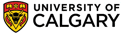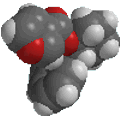Chapter 11 : Arenes and Aromaticity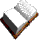Arenes and Aromaticity Questions
 Qu 1 Use the following list of compounds to answer the questions below: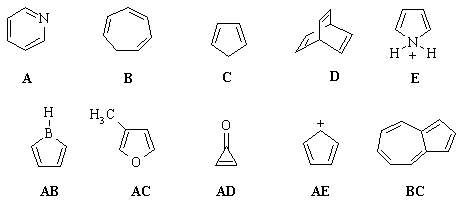Select the compound that is best described as: (a) A neutral, 4 p-electron, anti-aromatic system. (b) A 6 p-electron, aromatic system. (c) An aromatic system because n=2 in the Huckel 4n+2 rule. (d) A non-aromatic, conjugated 6 p-electron system (e) A non conjugated hydrocarbon. (f) Non-aromatic as drawn, but if H- were removed would give an aromatic cation. (g) Non-aromatic as drawn, but has an important resonance structure that is aromatic. (h) Non-aromatic as drawn but has an aromatic conjugate base. Qu 2: Consider the pKa data for the following aromatic N containing systems: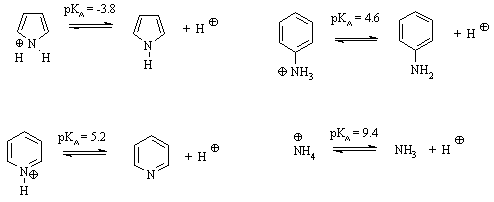Henderson-Hasselbach equation:      pKa = pH + log ([A-]/[HA]) Based on the scheme provided, (a) Which species is the strongest acid ? (b) At pH=4.2, what is the relative ratio of pyridine to its conjugate acid ? (c) Which is the better base:  pyridine or aniline, and why ? (d) Why is pyrrole is a weaker base than pyridine? Qu 3: Rate the resonance energies (in comparison to one another) of each of the following: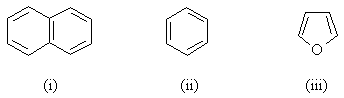Qu 4: Rate the relative stability of the following carbocations (in comparison to one another):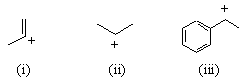Qu 5: Use the following data to answer the questions below: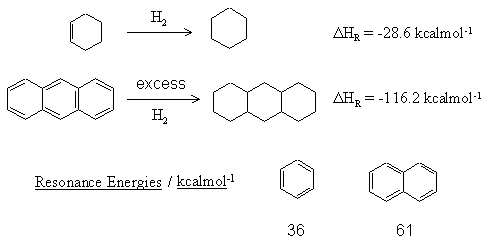(a) Calculate the resonance energy of anthracene,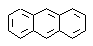(b) Anthracene is known to react with maleic anhydride in a Diels-Alder reaction. Predict which of the products shown  is obtained. Why ?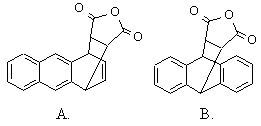© Dr. Ian Hunt, Department of Chemistry